# Part 3: Segmentation of an aorta¶

In the previous notebook, we presented the most fundamental operations of image processing. Let us now showcase their use on a simple toy problem: the segmentation of an aorta from axial slices.

As always, we first need to re-import the necessary modules and define our custom display routine:

In :
%matplotlib inline
import center_images             # Center our images
import matplotlib.pyplot as plt  # Graphical display
import numpy as np               # Numerical computations

def display(im):  # Define a new Python routine
"""
Displays an image using the methods of the 'matplotlib' library.
"""
plt.figure(figsize=(8,8))                    # Create a square blackboard
plt.imshow(im, cmap="gray", vmin=0, vmax=1)  # Display 'im' using gray colors,
#     from 0 (black) to 1 (white)


We will showcase our method on images kindly provided by Tom Boeken from the HEGP hospital - strictly following legal requirements and consent:

In :
# Import our images as grayscale, normalized from 0 (black) to 1 (white)
Images = [ imread("data/aortes/{}.jpg".format(i), as_gray=True) / 255
for i in [1,2,3,4,5,6] ]
# 'Images' is a list of six 2d arrays, denoted by 'Images', ..., 'Images'

plt.figure(figsize=(15,10)) # Rectangular blackboard
for i in [1,2,3,4,5,6] :    # Loop on indices i from 1 to 6
plt.subplot(2,3,i)      # Sub-plot "i" in a 2x3 waffle plot
plt.imshow( Images[i-1], cmap="gray", vmin=0, vmax=1) # Images is top-left, etc.
plt.title("Images[{}]".format(i-1))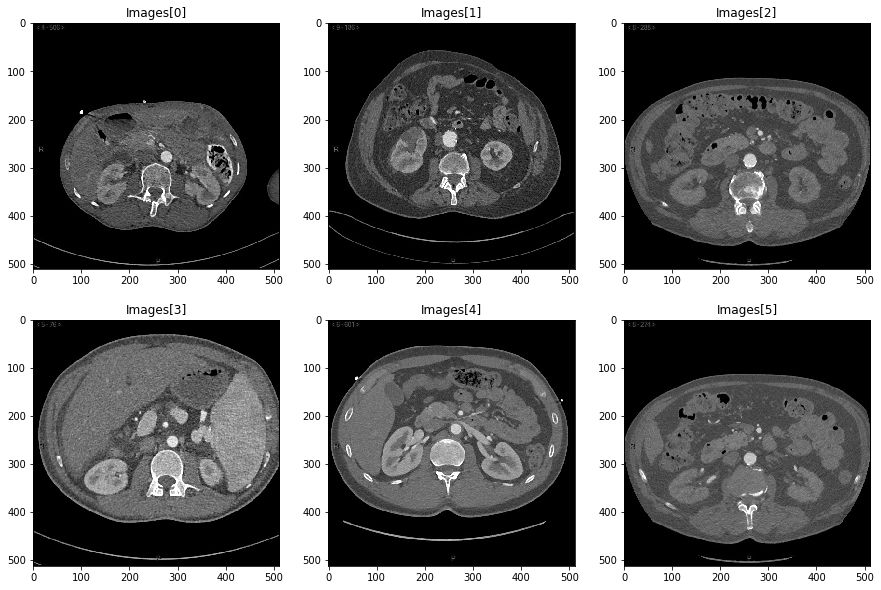First, we focus on the top-left slice and re-name it I:

In :
I = Images
display(I)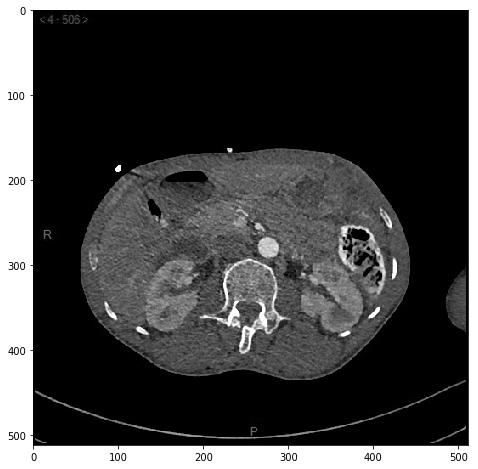How can we extract the aorta from the surrounding background? An acute human eye performs this "trivial" pre-processing step in a fraction of a second... But our computer cannot benefit from the machinery hardcoded in the human brain! One way or another, we must find a way to decompose this operation into simple, basic lines of code.

Fortunately, in this toy example, the color of the aorta is clearly different from that of the other tissues. Using a simple threshold, we should thus be able to make a huge step in the right direction:

In :
mask = (.6 < I) & (I < .9)  # Only keep pixel whose intensity lies in the (.6,.9) range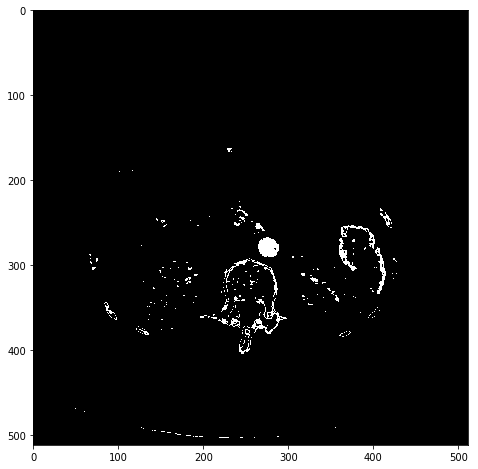Great! Our aorta is now the only structure that is both thick and bright. To extract it, we will use simply operations from mathematical morphology:

In :
from scipy.ndimage.morphology import binary_erosion  # "Erode" white structures
J = binary_erosion(mask)  # by removing pixels which have a black neighbour
display(J)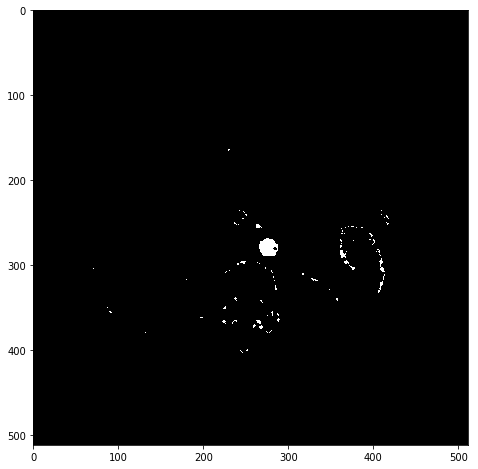In :
# Remove pixels which are close to the black background:
core = binary_erosion(mask, iterations=5)  # Iterate "erosion" five times
display(core)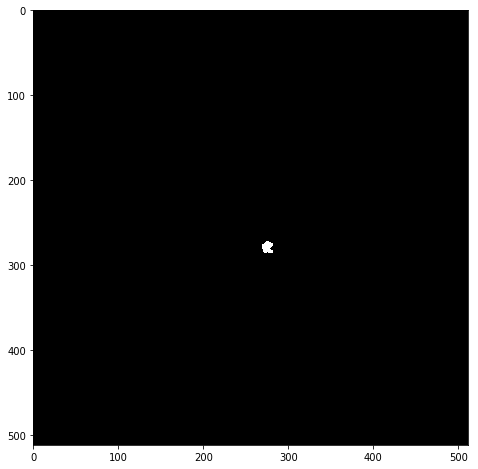A succession of thresholdings and erosions has allowed us to locate the core of our aorta. We can now let it grow to delimit a reasonable trust region:

In :
from scipy.ndimage.morphology import binary_dilation  # Invert "erosion":
region = binary_dilation(core, iterations=10)  # Let the "white region" grow 10 times
display(region)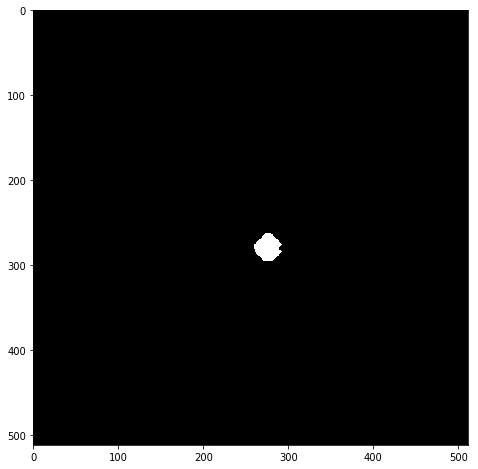And, finally, intersect this mask with the set of pixels "which are bright enough":

In :
aorta  = (I > .6) & region  # Intersection between "image is not too dark"
#                        and the trust region

# Fancy display in a 3x2 waffle grid ---------------------------------------------
plt.figure(figsize=(16,24))
plt.subplot(3,2,1); plt.imshow(I,         cmap="gray"); plt.title("Raw data")